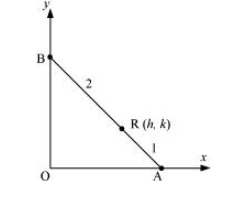# Point R (h, k) divides a line segment between the axes in the ratio 1:2.

Question:

Point R (h, k) divides a line segment between the axes in the ratio 1:2.  Find equation of the line.

Solution:

Let AB be the line segment between the axes such that point R (hk) divides AB in the ratio 1: 2.Let the respective coordinates of A and B be (x, 0) and (0, y).

Since point R (hk) divides AB in the ratio 1: 2, according to the section formula,

$(h, k)=\left(\frac{1 \times 0+2 \times x}{1+2}, \frac{1 \times y+2 \times 0}{1+2}\right)$

$\Rightarrow(h, k)=\left(\frac{2 x}{3}, \frac{y}{3}\right)$

$\Rightarrow h=\frac{2 x}{3}$ and $k=\frac{y}{3}$

$\Rightarrow x=\frac{3 h}{2}$ and $y=3 k$

Therefore, the respective coordinates of $A$ and $B$ are $\left(\frac{3 h}{2}, 0\right)$ and $(0,3 k)$.

Now, the equation of line AB passing through points $\left(\frac{3 h}{2}, 0\right)$ and

$(0,3 k)$ is

$(y-0)=\frac{3 k-0}{0-\frac{3 h}{2}}\left(x-\frac{3 h}{2}\right)$

$y=-\frac{2 k}{h}\left(x-\frac{3 h}{2}\right)$

$h y=-2 k x+3 h k$

i.e., $2 k x+h y=3 h k$

Thus, the required equation of the line is $2 k x+h y=3 h k$.